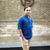# Toggle a boolean value (0 to 1 and 1 to 0) for selected features

513
3
07-16-2021 01:55 AMNew Contributor

Hello there,

I want to toggle line features between active and inactive. So I added a field "active" (integer) and set the namespace to 0, 1 (using a domain). Now I need to toggle this value using a shortcut.

For example: I select 10 Features and want to "invert the value" (0 to 1, 1 to 0).

Any Idea how to solve this?

1 Solution

Accepted SolutionsbyMVP Esteemed Contributor

Assuming there is no null value in the corresponding field, you may check the following expression using Calculate Field.

``IIF((\$feature.active == 0), 1,0)``

__________________________________________________________________________________________________

You could also calculate the field using Python parser.

Expression:

``Toggle(!active!)``

Code Block:

``````def Toggle(Bool):
if (Bool == 0):
return 1
else:
return 0``````

Think Location
3 RepliesbyMVP Esteemed Contributor

Assuming there is no null value in the corresponding field, you may check the following expression using Calculate Field.

``IIF((\$feature.active == 0), 1,0)``

__________________________________________________________________________________________________

You could also calculate the field using Python parser.

Expression:

``Toggle(!active!)``

Code Block:

``````def Toggle(Bool):
if (Bool == 0):
return 1
else:
return 0``````

Think LocationNew Contributor

Thanks, I know about "calculate field". Is it possible to run this function via keyboard shortcut? I`m looking for an easier way than open table, type expression and calculate field.

Best regards

PhilippbyMVP Esteemed Contributor

You may create a tool from Model Builder (use Calculate Field GP Tool) with predefined expression. Use the corresponding layer as input. Store the tool in the default toolbox of the project. You just need to run the tool each time you need to toggle the values.

Think Location